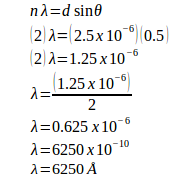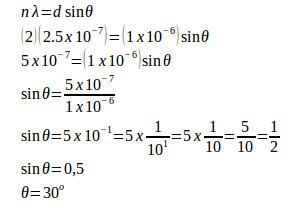# Diffraction grating – problems and solutions

1. A grating containing 4000 slits per centimeter is illuminated with a monochromatic light and produces the second-order bright line at a 30° angle. What is the wavelength of the light used? (1 Å = 10-10 m)

Known :

The distance between slits (d) = 1 / (4000 slits / cm) = 0.00025 cm = 2.5 x 10-4 cm = 2.5 x 10-6 meters

Order (n) = 2

Sin 30o = 0.5

1 Å = 10-10 m

Wanted: Wavelength of the light (λ)

Solution :[irp]

2. A monochromatic light with a wavelength of 2.5x10-7 m strikes a grating containing 10,000 slits/cm. Determine the angular positions of the second-order bright line.

Known :

The distance between slits (d) = 1 / (10,000 slits / cm) = 0.0001 cm = 1 x 10-4 cm = 1 x 10-6 m

Order (n) = 2

Wavelength (λ) = 2.5 x 10-7 m

Wanted : Angle (θ)

Solution :[irp]

3. A monochromatic light with wavelength of 5.10-7 m strikes a grating. Distance between slit and the viewing screen is 2 m, distance between the third-order fringe and the central fringe is 150 cm. Determine distance between slits.

Known :

Wavelength (λ) = 5 x 10-7 m

l = 2 m

n = 3

y = 150 cm = 1.5 m

Wanted : distance between slits

Solution :

sin θ ≈ tan θ = y / l = 1.5 / 2 = 0.75 = 75 x 10-2

Distance between slits :

d sin θ = n λ

d (75.10-2) = (3)(5.10-7)

d (75.10-2) = 15.10-7

d = (15.10-7) / (75.10-2)

d = (15/75).10-5

d = (1/5)(10-5) m

d = (0.2)(10-5) m

d = 2.10-6 m

4. A monochromatic light with wavelength of 500 nm (1 nano = 10-9) strikes a grating and produces the fourth-order bright line at an 30° angle. Determine the number of slits per centimeter.

Known :

Wavelength (λ) = 500.10-9 m = 5.10-7 m

θ = 30o

n = 4

Wanted : number of slits per centimeter

Solution :

Distance between slits :

d sin θ = n λ

d (sin 30o) = (4)(5.10-7)

d (0.5) = 20.10-7

d = (20.10-7) / 0.5

d = 40.10-7

d = 4.10-6 m

Number of slits per centimeter :

x = 1 / 4.10-6 m

x = 0.25.106 / m

x = 0.25.106 / 102 cm

x = 0.25.104 / cm

x = 25.102 / cm

x = 2500 / cm

5. A monochromatic light with wavelength of 500 nm (1 nm = 10-9 m) strikes a grating and produces the second-order bright line at an 30° angle. Determine the number of slits per centimeter.

Known :

Wavelength (λ) = 500.10-9 m = 5.10-7 m

θ = 30o

n = 2

Wanted : number of slits per centimeter

Solution :

Distance between slits :

d sin θ = n λ

d (sin 30o) = (2)(5.10-7)

d (0.5) = 10.10-7

d = (10.10-7) / 0.5

d = 20.10-7

d = 2.10-6 m

Number of slits per centimeter :

x = 1 / d

x = 1 / 2.10-6 m

x = 0.5.106 / 1 m

x = 0.5.106 / 102 cm

x = 0.5.104 / cm

x = 5.103 / cm

x = 5000 / cm